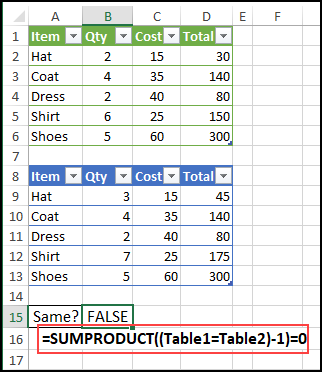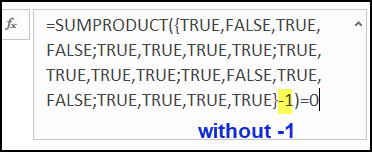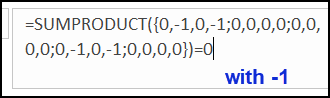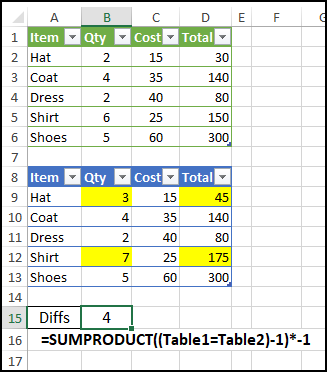## Compare Two Excel Tables with Simple Formula

Do you ever need to compare two Excel Tables? Here’s a simple formula that quickly shows if there are any differences, between tables that have the same number of columns and rows.

### Compare Two Tables

This simple, and very short, formula will compare two Excel Tables, that should be identical, but might have differences. Maybe one or two numbers have changed, but those little differences aren’t easy to see.

With this formula, you won’t have to manually comparing the tables, row by row. And you won’t need to create columns full of formulas to look for differences.

Thanks to UniMord for this tip!

### Simple Formula

Just enter this formula, somewhere on the worksheet, using the names of your tables, where I have Table1 and Table2.

=SUMPRODUCT((Table1=Table2)-1)=0

The formula result is TRUE, if the tables are exactly the same. If there are any differences, the result is FALSE.### SUMPRODUCT Comparisons

First, the SUMPRODUCT function multiplies the array of table value comparisons, and returns the sum.

SUMPRODUCT((Table1=Table2)-1)

Next, the formula tests to see if the sum is equal to zero.

=0

### Why Subtract 1?

Do you know why there is “-1” in the formula?

This formula compares each cell in the two tables. and we can see the array of results in the Formula Bar.

If you highlight just this part of the formula — (Table1=Table2) — in the Formula Bar, and press F9 to calculate, you’ll see all the results as TRUE or FALSE### Convert to Numbers

However, if you also include the -1, and the press F9 again, that arithmetic operation changes the results to numbers.If all the comparisons are TRUE (1),

• all the numbers will be zero (1-1)
• the total will be zero

If any comparisons are FALSE (0)

• those differences will show as -1 (0-1)
• the total will not be zero.

### How Many Differences?

To see how many differences there are between the two tables, you can make a slight change to the formula.

Instead of testing if the sum is equal to zero, multiply the sum by -1.

=SUMPRODUCT((Table1=Table2)-1)*-1

In this example, there are four differences between the two tables.### More SUMPRODUCT Examples

There’s lots that you can do with the under-appreciated SUMPRODUCT function. See more SUMPRODUCT examples on my Contextures website.

_________________

### Compare Two Excel Tables with Simple Formula_________________